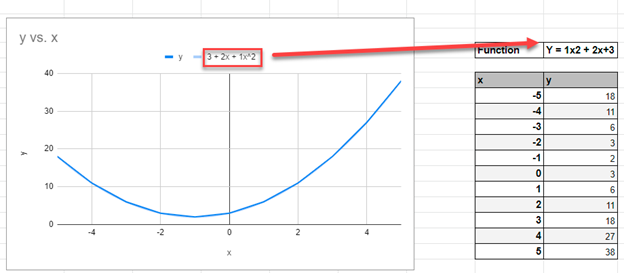# How to Graph an Equation / Function – Excel & Google Sheets

This tutorial will demonstrate how to graph a Function in Excel & Google Sheets.

## How to Graph an Equation / Function in Excel

1. Create the Function that you want to graph
2. Under the X Column, create a range. In this example, we’re range from -5 to 5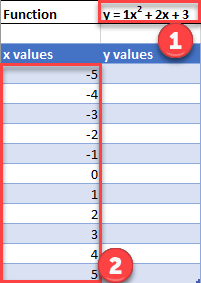### Fill in Y Column

Create a formula using the Function, substituting x with what is in Column B.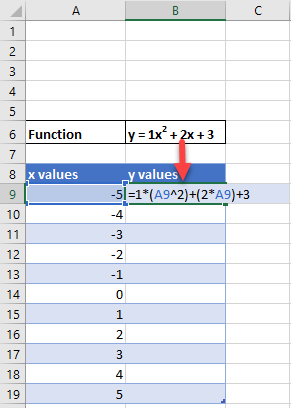After using this formula for all the rows, you should have a table that looks like below.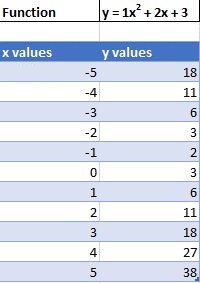### Creating Scatterplot

1. Highlight Dataset
2. Select Insert
3. Select Scatterplot
4. Select Scatter with Smooth Lines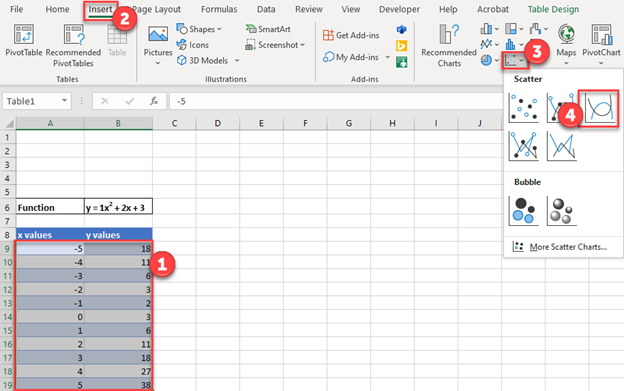This will create a graph that should look similar to below.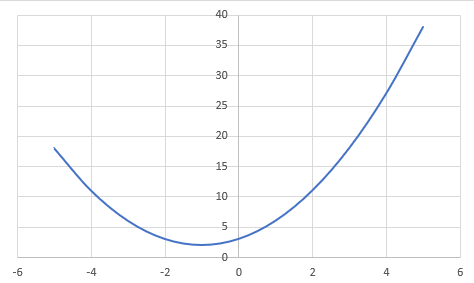### Add Equation Formula to Graph

1. Click Graph
2. Select Chart Design
4. Click Trendline
5. Select More Trendline Options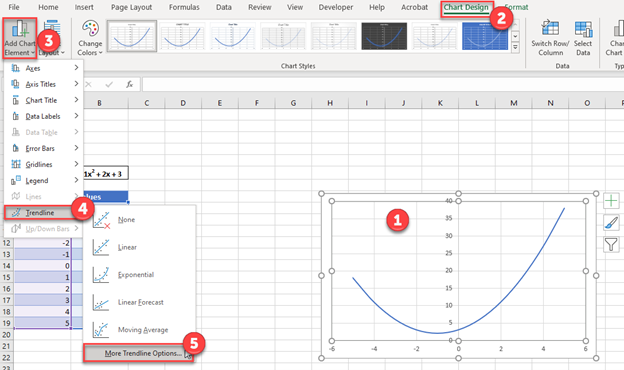6. Select Polynomial

7. Check Display Equation on Chart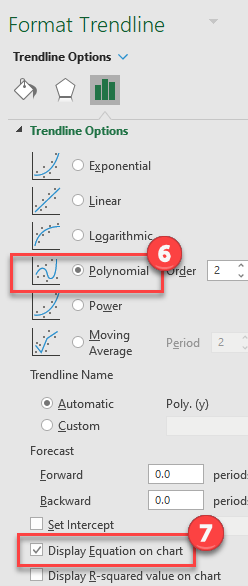### Final Scatterplot with Equation

Your final equation on the graph should match the function that you began with.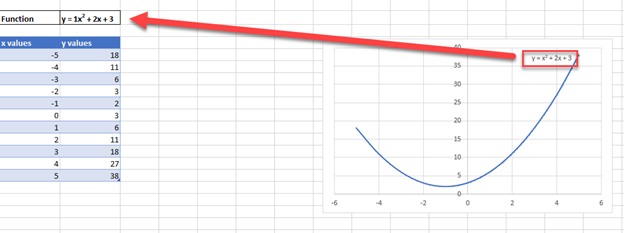### Easy Excel Automation## How to Graph an Equation / Function in Google Sheets

### Creating a Scatterplot

1. Using the same table that we made as explained above, highlight the table
2. Click Insert
3. Select Chart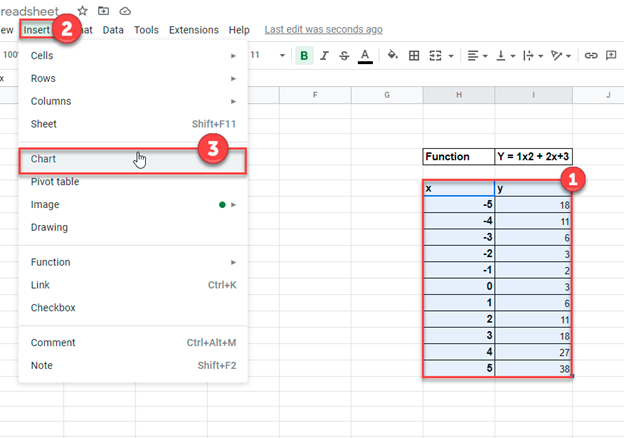4. Click on the dropdown under Chart Type

5. Select Line Chart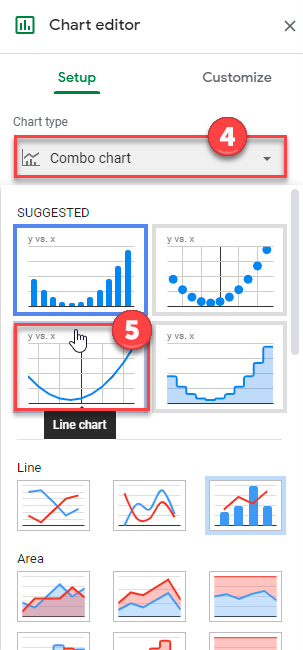1. Click on Customize
2. Select Series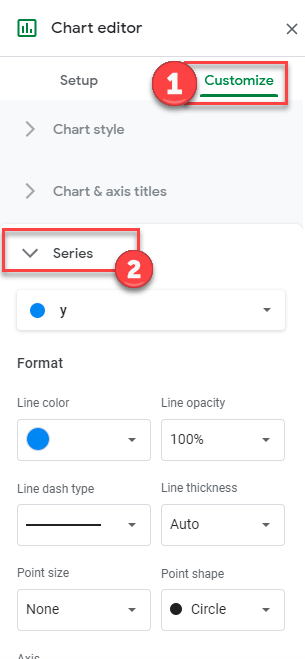3. Check Trendline

4. Under Type, Select Polynomial

5. Under Label, Select Use Equation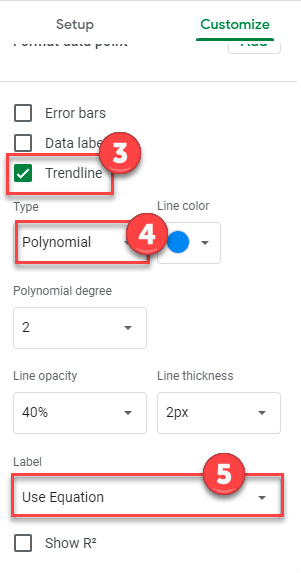### Final Scatterplot with Equation

As you can see, similar to the exercise in Excel, the equation matches the function that we began with.